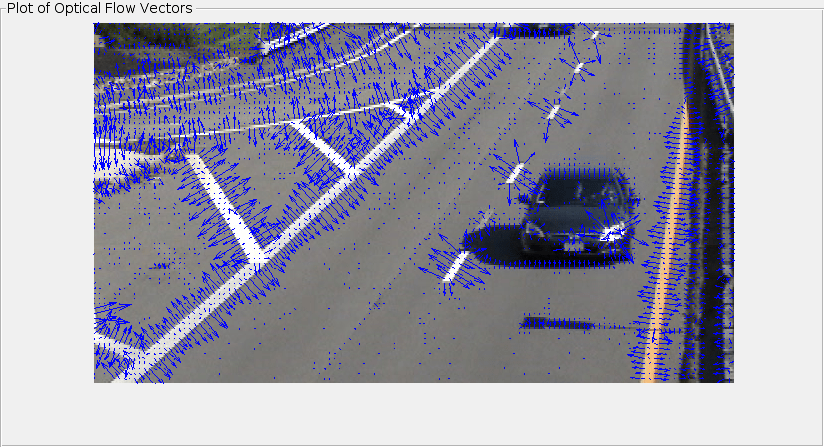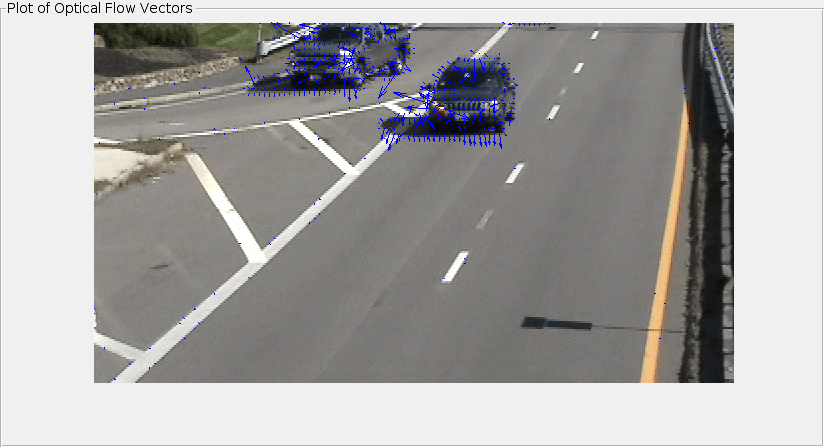# estimateFlow

Estimate optical flow

## Syntax

``flow = estimateFlow(opticFlow,I)``

## Description

example

````flow = estimateFlow(opticFlow,I)` estimates optical flow between two consecutive video frames. ```

## Examples

collapse all

Create a `VideoReader` object for the input video file, `visiontraffic.avi`. Specify the timestamp of the frame to read as 11.

`vidReader = VideoReader('visiontraffic.avi','CurrentTime',11);`

Specify the optical flow estimation method as `opticalFlowHS`. The output is an object specifying the optical flow estimation method and its properties.

`opticFlow = opticalFlowHS`
```opticFlow = opticalFlowHS with properties: Smoothness: 1 MaxIteration: 10 VelocityDifference: 0 ```

Create a custom figure window to visualize the optical flow vectors.

```h = figure; movegui(h); hViewPanel = uipanel(h,'Position',[0 0 1 1],'Title','Plot of Optical Flow Vectors'); hPlot = axes(hViewPanel);```

Read image frames from the `VideoReader` object and convert to grayscale images. Estimate the optical flow from consecutive image frames. Display the current image frame and plot the optical flow vectors as quiver plot.

```while hasFrame(vidReader) frameRGB = readFrame(vidReader); frameGray = rgb2gray(frameRGB); flow = estimateFlow(opticFlow,frameGray); imshow(frameRGB) hold on plot(flow,'DecimationFactor',[5 5],'ScaleFactor',60,'Parent',hPlot); hold off pause(10^-3) end```## Input Arguments

collapse all

Object for optical flow estimation, specified as one of the following:

The input `opticFlow` defines the optical flow estimation method and its properties used for estimating the optical flow velocity matrices.

Current video frame, specified as a 2-D grayscale image of size m-by-n. The input image is generated from the current video frame read using the `VideoReader` object. The video frames in RGB format must be converted to 2-D grayscale images for estimating the optical flow.

## Output Arguments

collapse all

Object for storing optical flow velocity matrices, returned as an `opticalFlow` object.

## Algorithms

The function estimates optical flow of the input video using the method specified by the input object `opticFlow`. The optical flow is estimated as the motion between two consecutive video frames. The video frame T at the given instant tcurrent is referred as current frame and the video frame T-1 is referred as previous frame. The initial value of the previous frame at time tcurrent = 0 is set as a uniform image of grayscale value 0.

### Note

If you specify `opticFlow` as `opticalFlowLKDoG` object, then the estimation delays by an amount relative to the number of video frames. The amount of delay depends on the value of `NumFrames` defined in `opticalFlowLKDoG` object. The optic flow estimated for a video frame at tcurrent corresponds to the video frame at time ${t}_{flow}=\left({t}_{current}-\left(NumFrames-1\right)/2\right)$. tcurrent is the time of the current video frame.